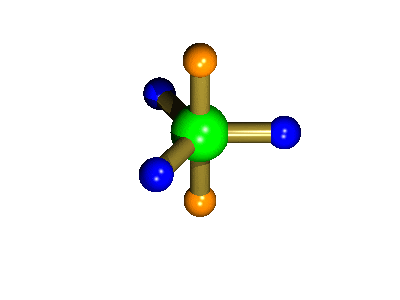DR ANTHONY MELVIN CRASTO,WorldDrugTracker, helping millions, A 90 % paralysed man in action for you, I am suffering from transverse mylitis and bound to a wheel chair, With death on the horizon, nothing will not stop me except God................DR ANTHONY MELVIN CRASTO Ph.D ( ICT, Mumbai) , INDIA 25Yrs Exp. in the feld of Organic Chemistry,Working for GLENMARK GENERICS at Navi Mumbai, INDIA. Serving chemists around the world. Helping them with websites on Chemistry.Million hits on google, world acclamation from industry, academia, drug authorities for websites, blogs and educational contribution

### Multinuclear NMR Spectroscopy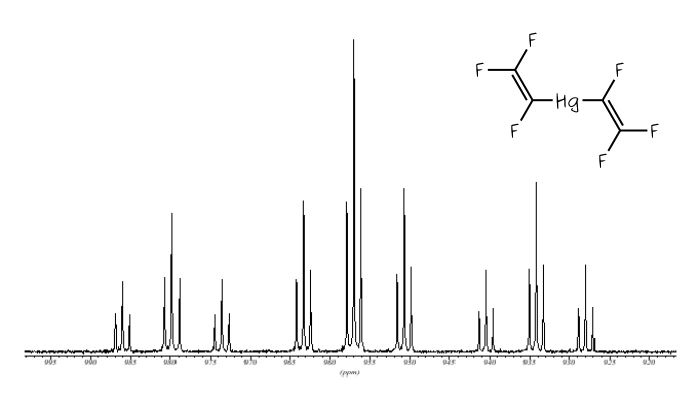.

examples of 19F, 31P, 77Se, 119Sn, 199Hg

## The 19F NMR spectra of C6F3H2Br.

The spectrum below was obtained for a sample of the partially fluorinated aromatic compound 1-bromo,3,4,5-trifluorobenzene. In this molecule there are two different chemical environments for the fluorine nuclei, that is the fluorine atoms in the 3 and 5 positions (blue in the diagram) are in one similar environment, while the C-4 fluorine (para to the bromine) is in its own unique environment. Consider either F-3 or F-5, this nucleus will couple with the adjacent F-4 nucleus, to give a doublet, and also with the neighbouring proton, which splits this signal again to generate a doublet of doublets. The single, unique fluorine, F-4, will couple with the two equivalent fluorine nuclei (F3 and F5) to give a triplet, and also with the two chemically equivalent protons so this signal appears as a triplet of triplets.

## The 19F NMR spectra of CF3CH2OCF2CF2H.

The spectrum below is for the fluoroether compound shown in the picture below. Because of free-rotation around the C-C bonds there are three different chemical environments for the fluorine nuclei; the CF3 group, the OCF2 fluorines and the CF2H fluorines. Consistent with this the spectrum shows 3 signals, in the relative intensity ratio (integrals) 3:2:2. The first of these signals, a ca. -75 ppm, is a triplet because of coupling to the adjacent 2 protons [2nI+1 => triplet]. The most negative signal, at ca. -137 ppm, is a doublet of triplets (see expansion) which arises from coupling to the singlet proton (to give a doublet) and then the two fluorine nuclei on the neighbouring carbon (to give the triplet part of the pattern) so generating a doublet of triplets overall. The middle signal arises from the two fluorines of the central OCF2 group.

## The 199Hg NMR spectra of Hg(CF=CF2)2 and Hg(CF=CF(CF3))2.

The spectrum below is of Hg(CF=CF2)2, also shown below. In this molecule all three fluorines of each trifluorovinyl group are chemically inequivalent to each other, but the same either side of the mercury centre, so the mercury signal is split into a triplet of triplet of triplets. [Click on the spectrum to see a larger version.]

The 199-Hg NMR spectrum shown below is for Hg(CF=CF(CF3))2. Compared with the molecule above, one of the fluorines of each group has been replaced by a CF3 group, so now the coupling pattern is a triplet of triplet of septets. However, the septet coupling is not very well resolved, even in the most intense, central portion of the spectrum the weakest outer lines (1:6:15:20:15:6:1) of the septet are difficult to pick out.

## The 31P and 19F NMR spectra of Ph2P(i-C3F7).

The spectra below are for a sample of Ph2P(i-C3F7). In this molecule there are two fuorine environments in the ratio 1:6, so the 31P NMR spectrum is a doublet of septets, as shown below. [Click on the spectrum to see a larger version.] However these are not completely separated, so there is some overlap in the centre of the pattern. The 19F NMR spectrum has two signals, reflecting the fact that there are two fluorine environments. The intense peak is due to the six fluorine nuclei of the two CF3 groups, which couple to the other fluorine, and the phosphorus to give a doublet of doublets. The weaker signal arises from the unique single fluorine environment, which shows coupling to the phosphorus and the six fluorines of the CF3 groups, so appears as a doublet of septets.

## 77Se NMR spectrum of Ph2P(Se)CH2CH2P(Se)Ph2.

The selenium NMR spectrum of the selenide of dppe bis(diphenylphospino)ethane is shown below. 77Se is of low abundance, only 7.6% (or 0.076), so there is the possibility of a molecule possessing either none, one or two Se-77 nuclei. The probability of these can be calculated in the following way:
```Neither selenium is 77: 0.924 x 0.924 = 0.854, or 85.4%
One selenium is 77:     2 x 0.076 x 0.924 = 0.140 or 14%
Both seleniums are 77 : 0.076 x 0.076 = 0.006 or 0.6%
```
Of course when neither selenium atom is 77Se there can be no 77-Se NMR spectrum. Conversely, the chance of both seleniums being 77Se is so low (0.6%) that we can effectively ignore it. This leaves us with the molecules in which one of the two seleniums is spin-active. In such molecules the two phosphorus atoms will be different - one is connected to a spin-active selenium, the other is not, so the selenium NMR signal is split into a widely separated doublet by the phosphorus nucleus to which it is directly bound (1J(SeP)) and also by the more distant, other phosphorus nucleus (4J(SeP)), so a doublet of doublets is observed, as shown below.

## Mutual Coupling - the 19F and 31P NMR spectra of K[PF6].

The spectra below were recorded for a solution of K[PF6] in DMSO. The six fluorines are all equivalent in the [PF6]- anion and so the 31P NMR spectrum consists of a septet. The separation between any adjacent pair of peaks is the P-F coupling, in this case 711 Hz. Since all six fluorine nuclei are equivalent they couple to just the phosphorus nucleus to give a doublet, again separated by 711 Hz.
Thus the two nuclei are said to exhibit mutual coupling, that is they couple to each other in the same way. The PF coupling constant labelled as 1J(PF) [where the superscript 1 refers to the 1 bond between the P and F nuclei] is 711 Hz. [Note: coupling constants are always quoted in Hz (which is independent of the spectrometer frequency) not ppm!]

## Satellites

Satellites are smaller peaks that appear either side of the main peak and arise because there is one or more non-100% abundant spin-active nucleus for a particular element. For example, the spectrum below is of Hg(CF3)2. It shows three peaks which arise as follows. Mercury has a number of different isotopes, one of these 199Hg is spin-active (I=1/2) but only 16.8% abundant. So of all the molecules of Hg(CF3)2, 83.2% (100% - 16.8%) will not contain spin-active mercury, and so the six equivalent fluorine nuclei will not couple to any other spin-active nuclei, so a singlet is observed. However, for the 16.8% of molecules containing 199Hg coupling will occur, so a doublet would arise (2nI+1 = 2). The actual spectrum observed is a combination of these two components - a singlet [83.2%] and a doublet [total intensity 16.8%, ie 8.4% each line.] with a similar chemical shift value, so one peak of the doublet appearing either side of the singlet.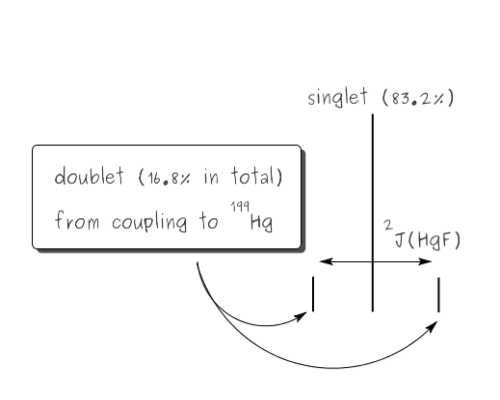The derivation of mercury satellites

The 2J(HgF) coupling constant is measured across the doublet, ie from one satellite peak to the other, in this case that is 1253 Hz. We can also check the relative intensities, since the signals due to 199Hg are the outer, weaker peaks, and these are (0.0990 + 0.0992) / (0.0990 + 0.999 + 0.0992) = 16.5% of the total.

## Berry pseudorotation

Compared with a number of other spectroscopic techniques NMR spectroscopy is relatively slow, so there is a chance that a molecule may be rearranging more quickly than the rate at which the spectroscopic process is occuring. If this happens then data for a time-averaged structure will be recorded.
Probably the most widely cited, inorganic, examples of such behaviour are the 5-coordinate molecules that adopt a trigonal bipyramidal structure, such as PF5. As shown below (left), these have (in a static structure) two environments, the two axial fluorines (orange) and three equatorial fluorines (blue). However, the 31P NMR spectrum of this molecule, recorded at room temperature, shows a sextet, suggesting that all 5 fluorines are equivalent. This occurs, because the molecule is in motion, via a square-based pyramidal intermediate (picture 2) that gives rise to an averaging, such that the axial and equatorial environments swap over (see picture 3).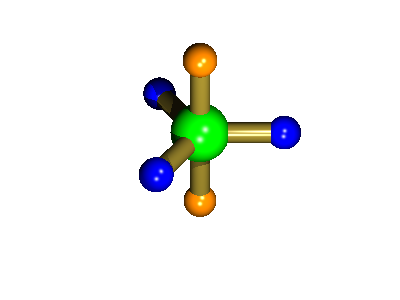Starting point, axial fluorine substituents are orange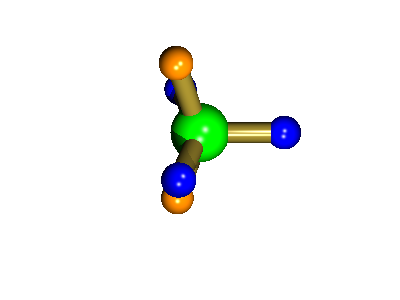The square-based pyramid intermediateOrange substituents are now equatorial
The whole of this process is illustrated below based on a POVRay generated movie. During the motion the two axial (orange) fluorines become equatorial, along with one of the blue atoms, while the other two blue coloured fluorines end up as the two axial substituents. In this way the positions of the fluorine atoms constantly change between being axial and equatorial, ie they become chemically equivalent.